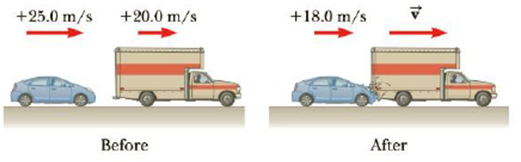Chapter 6, Problem 44P

Chapter
Section
Textbook Problem

A 1200-kg car traveling initially with a speed of 25.0 m/s in an easterly direction crashes into the rear end of a 9 000-kg truck moving in the same direction at 20.0 m/s (Fig. P6.44). The velocity of the car right after the collision is 18.0 m/s to the east, (a) What is the velocity of the truck right after the collision? (b) How much mechanical energy is lost in the collision? Account for this loss in energy.Figure P6.44

(a)

To determine
The final velocity of the truck.

Explanation

Given Info: Mass of the car is mC = 1200 kg, mass of the truck is mT = 9000 kg, initial velocity of the car is viC = 25.0 m/s , initial velocity of the truck is viT = 20.0 m/s and final  velocity of the car is vfC = 18.0 m/s.

Explanation:

Apply conservation of momentum to car-truck system is,

mTvfT+mCvfC=mTviT+mCviC (1)

• mC is the mass of the car.
• mT is the mass of the truck.
• viC is the initial velocity of the car.
• viT is the initial velocity of the truck.
• vfC is the final velocity of the car.

Substitute 1200kg for mC , 9000kg for mT , 25.0m/s for viC , 20

(b)

To determine
The loss of mechanical energy during collision.

Still sussing out bartleby?

Check out a sample textbook solution.

See a sample solution

The Solution to Your Study Problems

Bartleby provides explanations to thousands of textbook problems written by our experts, many with advanced degrees!

Get Started

A B vitamin often forms part of an enzyme's active site, where a chemical reaction takes place. T F

Nutrition: Concepts and Controversies - Standalone book (MindTap Course List)

What heats the chromospheres and corona to a high temperature?

Horizons: Exploring the Universe (MindTap Course List)

What is a peptide bond, and what type of reaction forms it?

Biology: The Dynamic Science (MindTap Course List)